VHDL coding tips and tricks: Fixed Point Operations in VHDL : Tutorial Series Part 3

## Monday, March 29, 2010

### Fixed Point Operations in VHDL : Tutorial Series Part 3

This article is a continuation of the tutorial series on fixed_pkg library.In this article I will talk about,arithmetical operations on fixed point signals.I assume that you have read Part 1 and Part 2 of the series.

If you have gone through Part 2 of the series then you must have seen that assigning a signal results in rounding off the value if the range of the output signal is not sufficient.Yes,this is a great advantage of fixed_pkg.It is designed so that there is no possibility of an overflow.This is different from "numeric_std" library which simply neglect the underflow and overflow bits.In order to make sure that overflow doesn't happen we have to carefully design the size of output signals based on input signal size and the operation performed.The following table shows the Results range for different kind of operations:

In the table A'left means the left most array index and A'right means the right most array index.The functions max and min are defined as follows:

max(a,b) = a if a > b else b.
min(a,b) = a if a < b else b.

The operations supported by the package for unsigned types are:
+, –, *, /, rem, mod, =, /=, <, >, >=, <=, sll, srl, rol, ror, sla, sra.

The operations supported by the package for signed types are:
+, –, *, /, rem, mod, =, /=, <, >, >=, <=, sll, srl, rol, ror, sla, sra, abs, - (unary).

Without going much into the explanations I will directly explain their use with the help of some examples.

Examples for addition:
signal n1,n2 : ufixed(4 downto -3);
signal n3 : ufixed(5 downto -3);
begin
n1 <=  to_ufixed (5.75,n1);     -- n1 = "00101110" = 5.75
n2 <=  to_ufixed (6.5,n2);      -- n2 = "00110100" = 6.5
n3 <= n1+n2;                   -- n3 = "001100010" = 12.25
--See that the range of 'n3' is 5 downto -3 but that of 'n1' and 'n2' is 4 downto -3.

If the range of n3 is 4 downto -3 then the result will be:
n3 = "00110001" = 6.125.
So it is very important to declare the output signal ranges as per the above table.

--For signed numbers:
signal s1,s2 : sfixed(4 downto -3);
signal s3 : sfixed(5 downto -3);
s1 <=  to_sfixed (5.75,s1);     -- s1 = "00101110" = 5.75
s2 <=  to_sfixed (-6.5,s2);     -- s2 = "11001100" = -6.5
s3 <= s1+s2;                   -- s3 = "111111010" = -0.75

Examples for Subtraction:
n1 <=  to_ufixed (5.75,n1);      -- n1 = "00101110" = 5.75
n2 <=  to_ufixed (6.5,n2);       -- n2 = "00110100" = 6.5
n3 <= n2-n1;                    -- n3 = "000000110" = 0.75

s1 <=  to_sfixed (5.75,s1);      -- s1 = "00101110" = 5.75
s2 <=  to_sfixed (-6.5,s2);      -- s2 = "11001100" = -6.5
s3 <= s2-s1;                    -- s3 = "110011110" = -12.25

Examples for multiplication:
signal n1,n2 : ufixed(4 downto -3);
signal n3 : ufixed(9 downto -6);

signal s1,s2,s3 : sfixed(4 downto -3);
signal s4,s5 : sfixed(9 downto -6);

n1 <=  to_ufixed (5.75,n1);         -- n1 = "00101110" = 5.75
n2 <=  to_ufixed (6.5,n2);          -- n2 = "00110100" = 6.5
n3 <= n2*n1;                        -- n3 = "0000100101011000" = 37.375

s1 <=  to_sfixed (5.75,s1);         -- s1 = "00101110" = 5.75
s2 <=  to_sfixed (-6.5,s2);         -- s2 = "11001100" = -6.5
s4 <= s2*s1;                        -- s4 = "1111011010101000" = -37.375

s3 <=  to_sfixed (-5.75,s1);        -- s3 = "11010010" = -5.75
s5 <= s3*s2;                        -- s5 = "0000100101011000" = 37.375

Examples for Remainder:
signal n1 : ufixed(4 downto -3);
signal n2 : ufixed(2 downto -4);
signal n3 : ufixed(2 downto -3);     -- see how range of n3 is declared here.

signal s1 : sfixed(4 downto -3);
signal s2 : sfixed(2 downto -4);
signal s3 : sfixed(2 downto -3);     -- see how range of s3 is declared here.

n1 <=  to_ufixed (7.25,n1);        -- n1 = "00111010" = 7.25
n2 <=  to_ufixed (1.5,n2);         -- n2 = "0011000" = 1.5
n3 <= n1 rem n2;                   -- n3 = "001010" = 1.25

s1 <=  to_sfixed (-7.25,s1);       -- s1 = "11000110" = -7.25
s2 <=  to_sfixed (-1.5,s2);        -- s2 = "1101000" = -1.5
s3 <= s1 rem s2;                   -- s3 = "110110" = -1.25

Examples for division:
signal n1 : ufixed(4 downto -3);
signal n2 : ufixed(2 downto -4);
signal n3 : ufixed(8 downto -6);     -- see how range of n3 is declared here.

signal s1 : sfixed(4 downto -3);
signal s2 : sfixed(2 downto -4);
signal s3 : sfixed(9 downto -5);     -- see how range of s3 is declared here.

n1 <=  to_ufixed (6.75,n1);          -- n1 = "00110110" = 6.75
n2 <=  to_ufixed (1.5,n2);           -- n2 = "0011000" = 1.5
n3 <= n1 / n2;                       -- n3 = "000000100100000" = 1.5

s1 <=  to_sfixed (-6.75,s1);         -- s1 = "11001010" = -6.75
s2 <=  to_sfixed (1.5,s2);           -- s2 = "0011000" = 1.5
s3 <= s1 / s2;                       -- s3 = "111111101110000" = -1.5

These are only a few of the operators available in the package.In the next part of the tutorial, I will explain the use of some more operators with example.
Read Part 1 and Part 2 of the series here:
Fixed Point Operations in VHDL : Tutorial Series Part 1
Fixed Point Operations in VHDL : Tutorial Series Part 2

#### 23 comments:

1.This tutorial was inspired from fixed_pkg" documentation by David Bishop.The original document can be downloaded from
http://www.vhdl.org/fphdl/Fixed_ug.pdf

2.how to implement qr decomposition matrix method in vhdl

3.This comment has been removed by the author.

4.When I try to execute de division I get the error, Found 0 definitions for operator "/".?

How can I solve it?

Thanks in advance

5.the result of devision is 4.5
thank you

6.Can I multi play a sfixed with integer type and store the results I a sfixed type?

7.This informations are very useful. I have 2 questions.

1) At ufixed rules table, A rem B has a boundary which is "min(A'left,B'left) downto min(A'right,B'right)". Is it true by looking your codes about rem operation? So to say that is like that "min(A'left,B'left) downto max(A'right,B'right)".

2)Is it possible to convert u/sfixed numbers to integer like that "to_integer(s1)".

8.I can't get the division example to work:
"/ can not have such operands in this context."
This is in the Xilinx ISE.

1.the code / should work in simulation level. It will not work if you are trying to synthesis the design.

2.I suspect it is trying to synthesise it first, thanks for the heads up.

9.what is libraries used in these examples (for signed numbers)

10.I'm trying to wrap my head around how maths works in vhdl and am struggling, I'm hoping someone can help with a little problem I'm having. I have a 16 bit ufixed value which I want to subtract an offset (another 16 bit ufixed), then multiply it by another ufixed. This is the relevant part of my logic:

constant conv_uf : ufixed (0 downto -15) := x"C800"; -- =1.5625, this the the multiply part
constant OFFSET :ufixed (15 downto 0) := x"2000"; -- =8192, the offset I want to subtract
signal Speed := STD_LOGIC_VECTOR (15 downto 0); -- this is my output vector
signal mA_uf := ufixed (15 downto 0); -- this is my input ufixed

if mA_uf > OFFSET -- check that we can subtract the OFFSET without going through zero
-- this is the troublesome line
Speed <= std_logic_vector (resize(conv_uf * (mA_uf - OFFSET), Speed'high, Speed'low));
else
Speed <= x"0000";
end if;

It simulates fine in Xilinx ISim, but in an FPGA is gives FFFF when mA_uf > OFFSET (for example, mA_uf := x"2001" gives FFFF). There is a bit more code which checks for the condition where the multiplication would result in >FFFF, but for simplicity I have reduced the code here to the minimum workable.

If someone can point me in the right direction it would be appreciated.

11.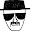i would try separating the sum first (mA_uf - OFFSET) into a variable with size Speed'high+1 down to Speed'low. Then for the multiply part have range (Speed'high+1)+1 downto conv_uf'low.

1.Much appreciated :)

12.I want to add three floating point no.Can you tell how the result range will vary.

13.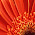I am trying to multiply an unsigned number with sfixed number (0.703125). following are the code and errors:

library IEEE;
use IEEE.STD_LOGIC_1164.ALL;
library ieee_proposed;
use ieee_proposed.fixed_pkg.all;
USE ieee.numeric_std.all;

entity tryproduct is
end tryproduct;

architecture Behavioral of tryproduct is (Line 11)
signal a : sfixed(0 downto -6);
signal k : sfixed(8 downto -6);
SIGNAL temp1: UNSIGNED (8 downto 0) := "101001001" ;

begin

a <= to_sfixed (0.703125,a);
k <= to_sfixed(temp1) * a; (Line 19)
end Behavioral;

Errors:
ERROR:HDLCompiler:432 - "G:/vhdlcodes/producttry/tryproduct.vhd" Line 19: Formal has no actual or default value.
ERROR:HDLCompiler:841 - "G:/vhdlcodes/producttry/tryproduct.vhd" Line 19: Expecting type integer for .
ERROR:HDLCompiler:9 - "G:/vhdlcodes/producttry/tryproduct.vhd" Line 19: Found 0 definitions for operator "*".
ERROR:HDLCompiler:854 - "G:/vhdlcodes/producttry/tryproduct.vhd" Line 11: Unit ignored due to previous errors.

thanks in advance

1.This bit of line 19:
to_sfixed(temp1)
doesn't specify the limits. I think you need to extend upper limit by 1 to accommodate the positive leading '0'. I'd try:
to_sfixed(temp1, 9, 0)
Also, the upper limit for k looks too low even without extending the upper limit of the temp1 conversion. I'd use:
signal k : sfixed(10 downto -6);

14.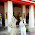The download link for the library seems to be down, do you have another link?
Thanks!

15.Send a mail to shp.ece@gmail.com I ll send the libraries to ur mail

16.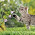please tell me how i can get the Library ieee_proposed??And with anything that can be replaced???

1.Why do you want to replace the libraries? These libraries come inbuilt with the tool. you dont have to separately download them.

17.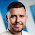Hello, i need a create program in VHDL : Fixed point multiplication integer. Can help me somebody ? I can send money for this.

18.yes. I can help for a fee. Send assignment details to this email, lalnitt (at) gmail (dot) com.